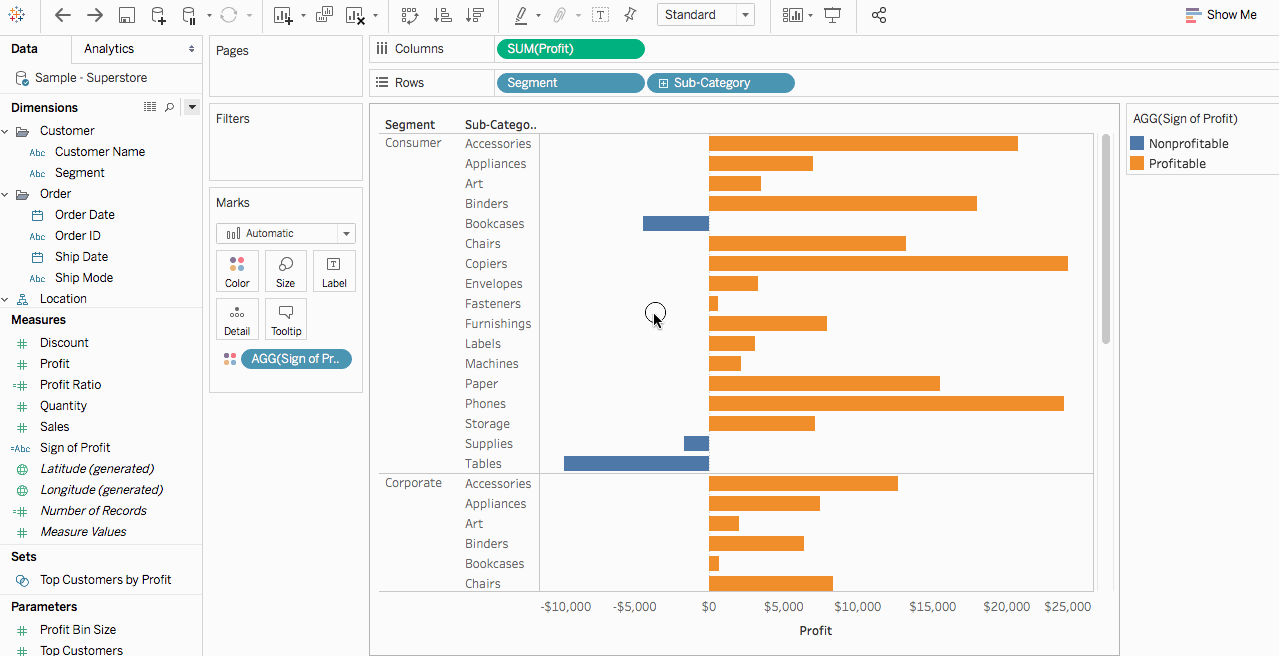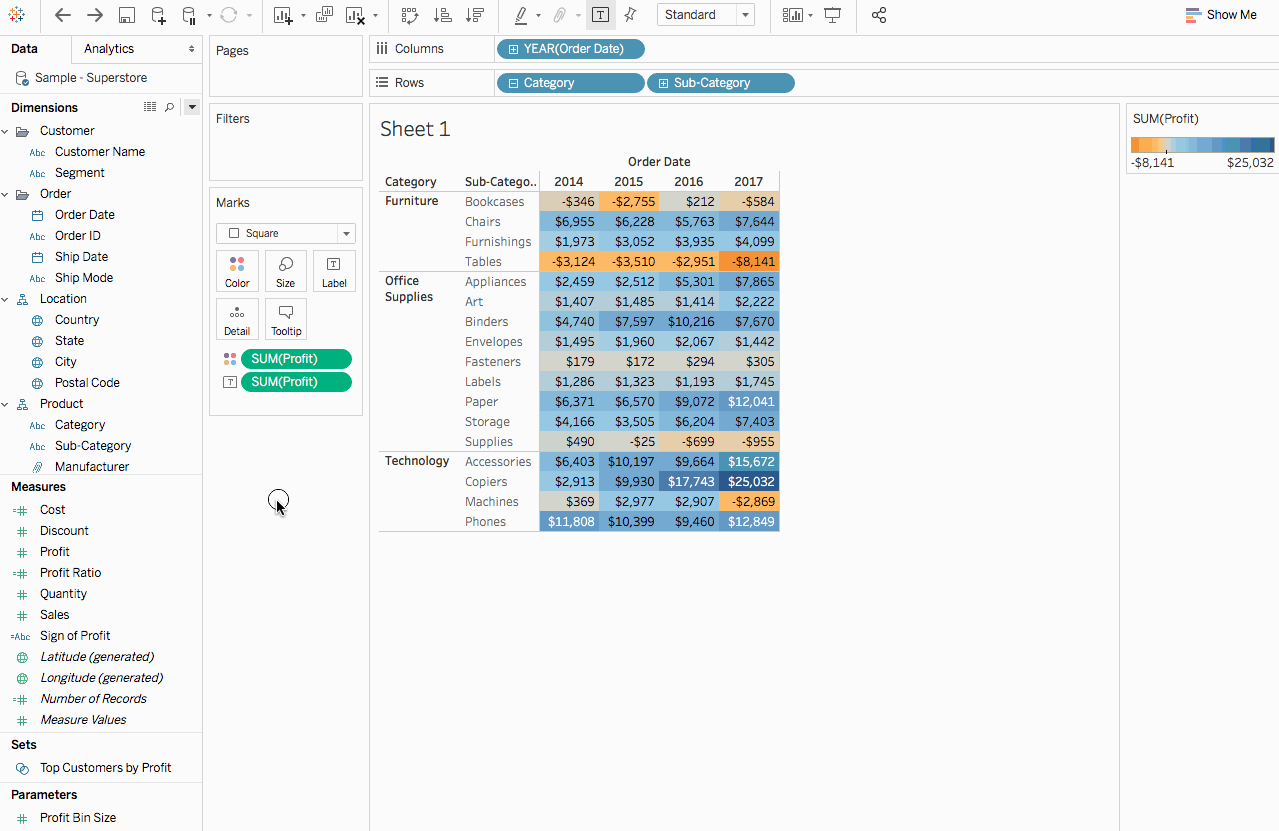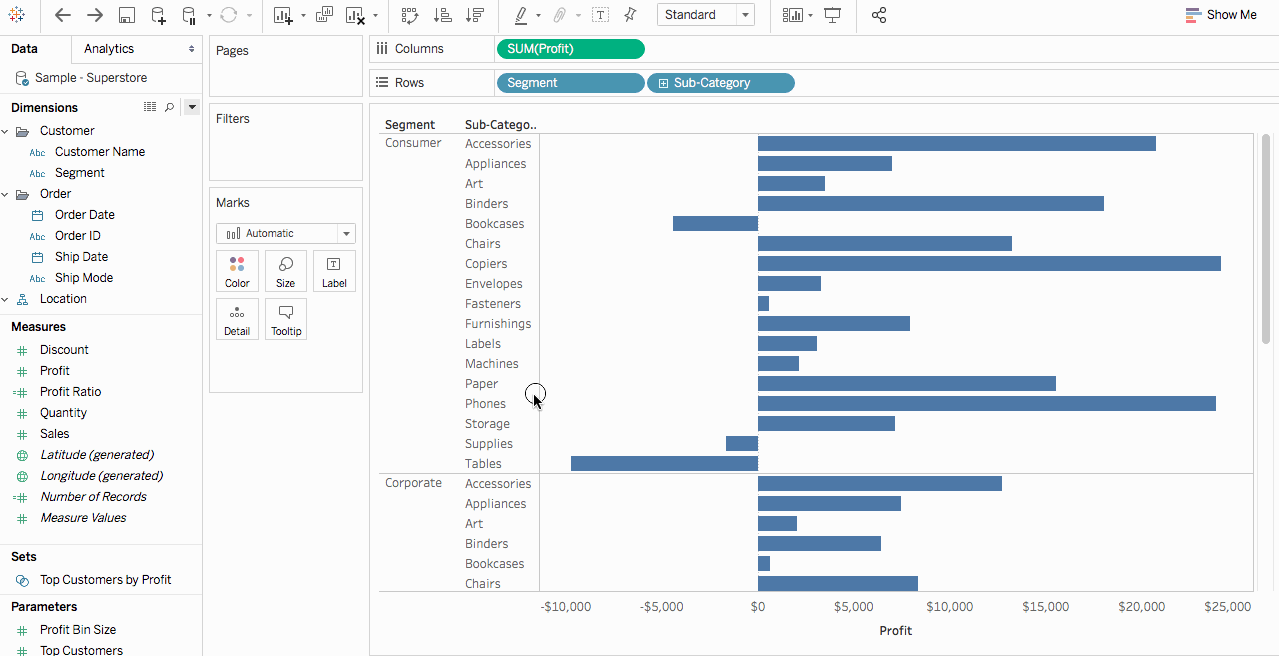# Understanding Calculations in Tableau

This series introduces the basics of understanding calculations in Tableau. In this topic, you'll learn why and when to use calculations.

## Why use calculations

Calculations allow you to create new data from data that already exists in your data source, as well as perform computations on your data. This allows you to perform complex analyzes and add fields to your data source on your own and on the fly.

## When to use calculations

The first hurdle to learning calculations in Tableau is to recognize when you actually need to use one. You can use calculations for many, many reasons. Some examples might include:

• To segment data
• To convert the data type of a field, such as converting a string to a date.
• To aggregate data
• To filter results
• To calculate ratios

Some common scenarios might include:

• The data you need for your analysis is missing from your data source.

For example, if you have a Sales and Profit field in your data source, but you want to calculate cost, you can create a Cost field using a formula similar to the following.

`[Sales] - [Profit]`• You want to transform values in your visualization.

For example, you might want to calculate the difference in profit from one year to the other. You can use a quick table calculation to show the difference in profit in the visualization.• You want to quickly categorize data.

For example, you might want to quickly color the data in your visualization as profitable or nonprofitable. You can create a calculated field using a calculation similar to the following and then add it to Color on the Marks card.

`IF SUM([Profit]) > 0THEN "Profitable" ELSE "Nonprofitable" END`